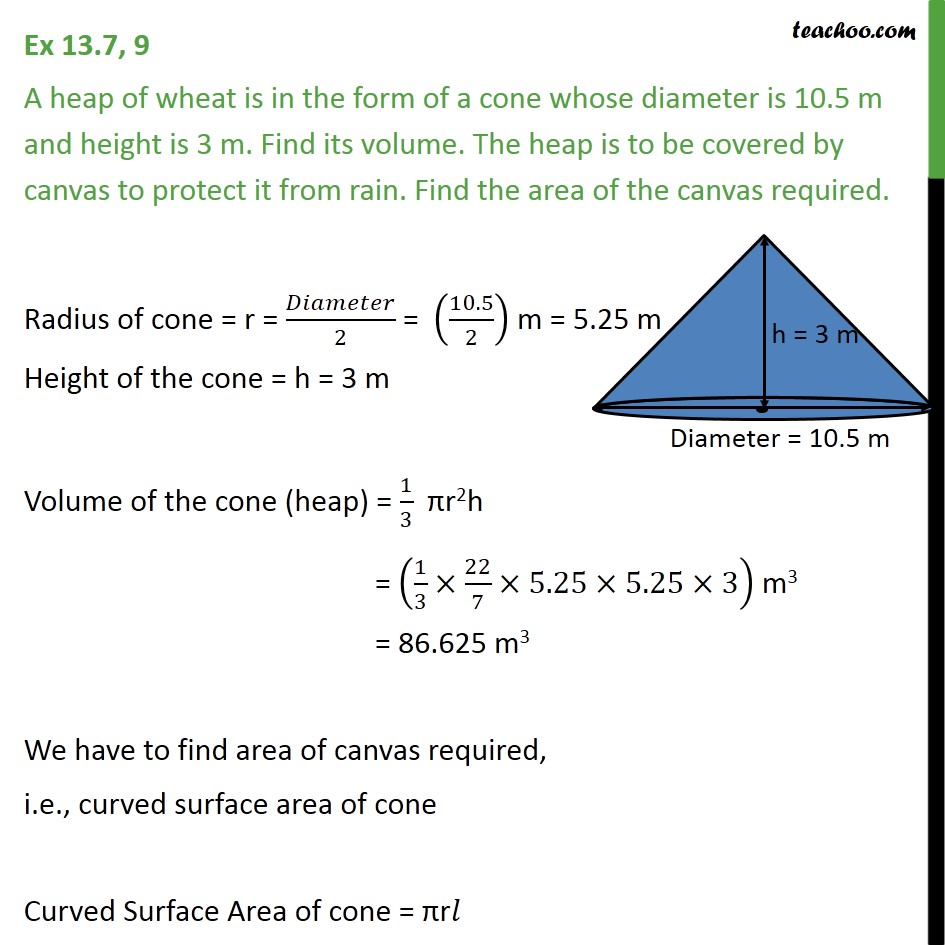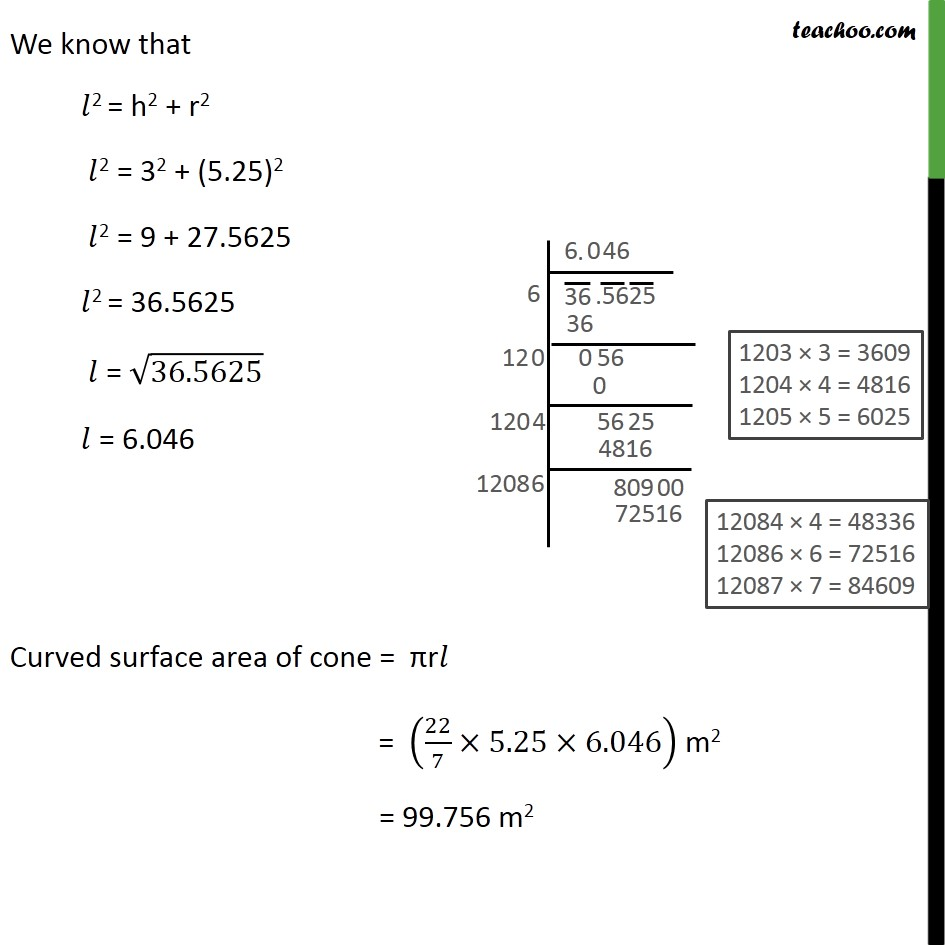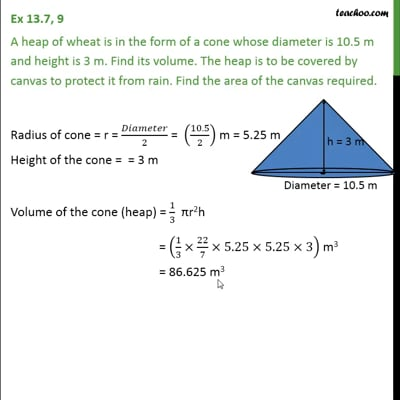Volume Of Cone

Chapter 13 Class 9 Surface Areas and Volumes
Concept wiseThis video is only available for Teachoo black users

Introducing your new favourite teacher - Teachoo Black, at only ₹83 per month

### Transcript

Ex 13.7, 9 A heap of wheat is in the form of a cone whose diameter is 10.5 m and height is 3 m. Find its volume. The heap is to be covered by canvas to protect it from rain. Find the area of the canvas required. Radius of cone = r = /2 = (10.5/2) m = 5.25 m Height of the cone = h = 3 m Volume of the cone (heap) = 1/3 r2h = (1/3 22/7 5.25 5.25 3) m3 = 86.625 m3 We have to find area of canvas required, i.e., curved surface area of cone Curved Surface Area of cone = r We know that 2 = h2 + r2 2 = 32 + (5.25)2 2 = 9 + 27.5625 2 = 36.5625 = 36.5625 = 6.046 Curved surface area of cone = r = (22/7 5.25 6.046) m2 = 99.756 m2